JUST position the first vector &

Example 1

Add vector a  to vector b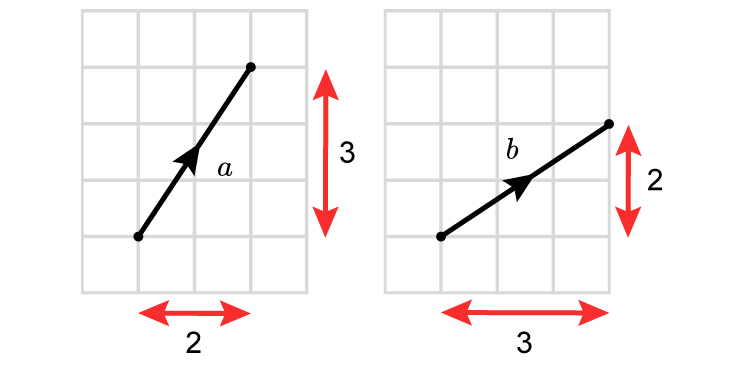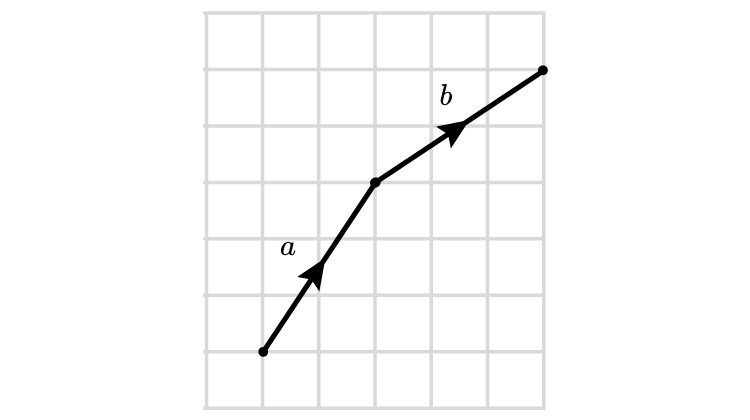Place the first vector a  and add on the second vector.

The result is a new vector c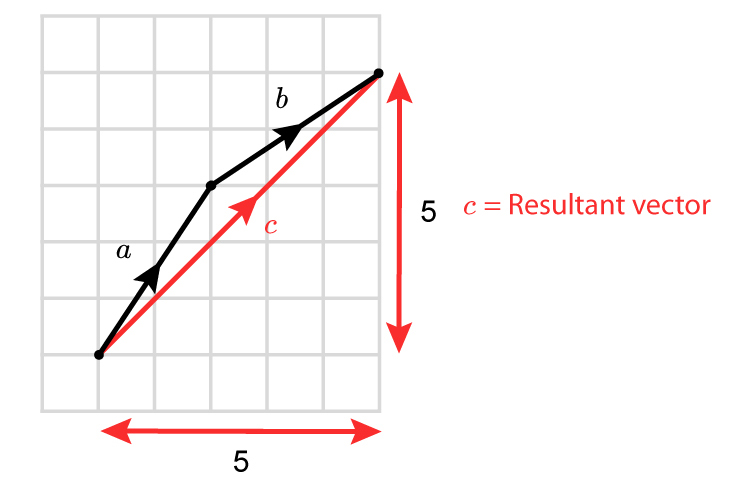NOTE:

It doesn't matter in which order you add the vectors.

Try adding b+a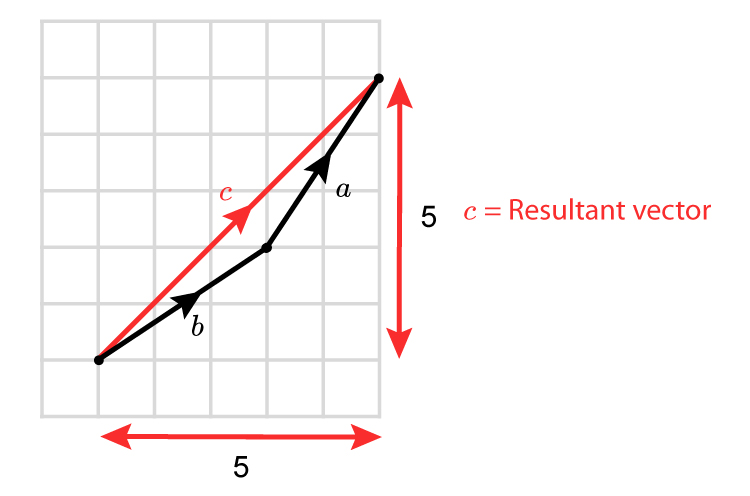a+b=c  and b+a=c

ANOTHER way of showing that a+b=b+a  is as follows:

Example 2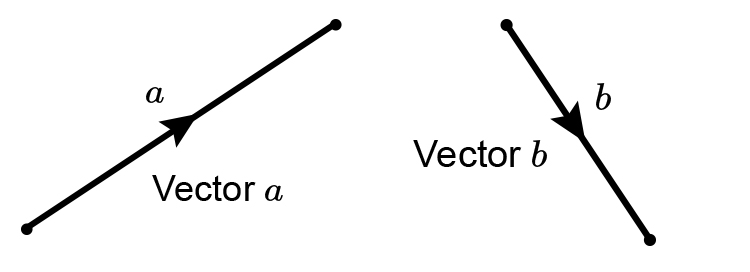a+b=b+a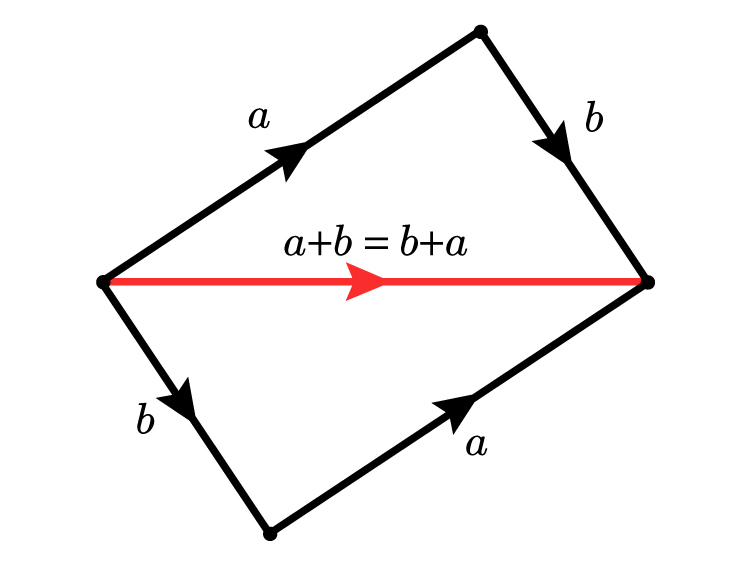Example 3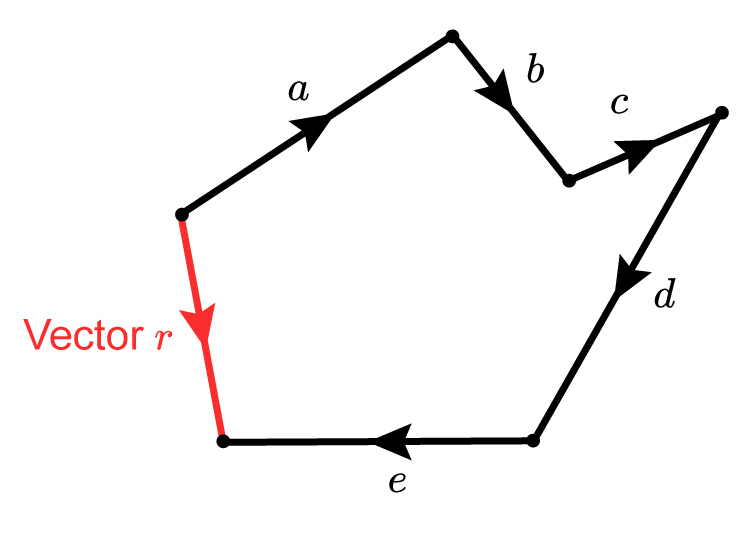Vector r=a+b+c+d+e

Also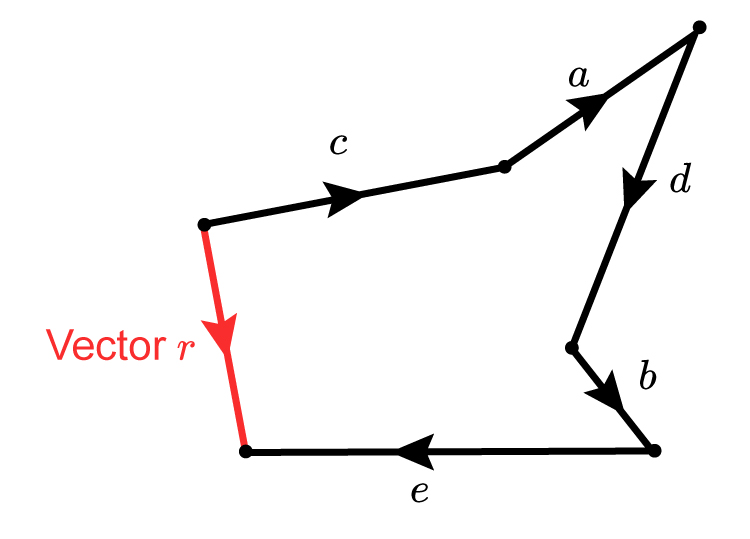Vector r=c+a+d+b+e

And also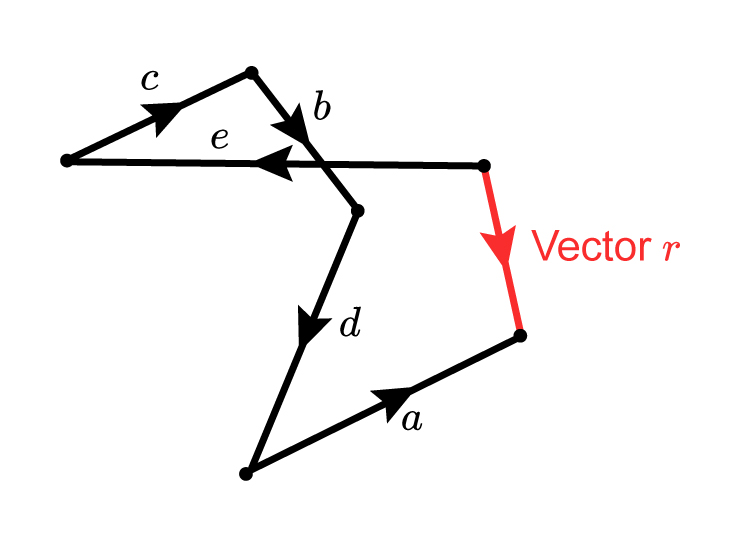Vector r=e+c+b+d+a

You can ADD IN ANY ORDER.